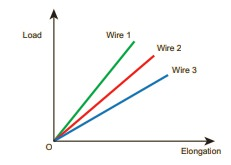Home | Multiple Choice Questions - Physics: Properties of Matter

# Multiple Choice Questions - Physics: Properties of Matter

1 mark questions and answers, Multiple Choice Questions - Physics: Properties of Matter

Properties of Matter (Physics)

Multiple Choice Questions

1. Consider two wires X and Y. The radius of wire X is 3 times the radius of Y. If they are stretched by the same load then the stress on Y is

(a) equal to that on X

(b) thrice that on X

(c) nine times that on X

(d) Half that on X

Answer : c) nine times that on X

Solution:

Žāx ŌłØ 1 / rx;

Žāy ŌłØ 1 / ry2

Žāx / Žā= (ry / r)= (ry / 3r)2

Žāx / Žā= (1/3)2 = 1 / 9

Žāy = 9 Žāx

2. If a wire is stretched to double of its original length, then the strain in the wire is

(a) 1

(b) 2

(c) 3

(d) 4

Solution:

╬Ą = ╬öl / l

╬Ą = [ lŌĆō lo ] / lo

= (2 ŌĆō 1) / 1 = 1 / 1 = 1

3. The load ŌĆō elongation graph of three wires of the same material are shown in figure. Which of the following wire is the thickest?(a) wire 1

(b) wire 2

(c) wire 3

(d) all of them have same thickness

Solution:

If slope is high the elongation will be less

4. For a given material, the rigidity modulus is (1/3) rd of YoungŌĆÖs modulus. Its PoissonŌĆÖs ratio is

(a) 0

(b) 0.25

(c) 0.3

(d) 0.5

Solution:

╬Ę = 1/3 y

y = 2 ╬Ę (1 + ╬╝ )

y = 2y/3 (1 + ╬╝ )

3/2 = 1 + ╬╝

╬╝ = (3/2) ŌĆō 1 = 1 / 2

╬╝ = 0.5

5. A small sphere of radius 2cm falls from rest in a viscous liquid. Heat is produced due to viscous force. The rate of production of heat when the sphere attains its terminal velocity is proportional to

(a) 22

(b) 23

(c) 24

(d) 25

Solution:

dQ / dt = E / t = Power

Power = P = F ├Ś vt

F = 6ŽĆ╬Ęrvt

dQ / dt = 6ŽĆ╬Ęrvt2

vt = [2/9] [ (Žü ŌĆō Žā ) / ╬Ę]g ├Ś [ r2]

dQ / dt = 6ŽĆ╬Ęr ├Ś ( 2/9 [ (ŽüŌĆōŽā)/╬Ę ├Ś g ])2 ├Ś r4

dQ / dt ŌłØ r5

6. Two wires are made of the same material and have the same volume. The area of cross sections of the first and the second wires are A and 2A respectively. If the length of the first wire is increased by Ōłål on applying a force F, how much force is needed to stretch the second wire by the same amount?

(a) 2

(b) 4

(c) 8

(d) 16

Solution:

y = Fl / [╬öl ├Ś A]

y = [ Fl / A╬öl ] ├Ś [ A ] = [F.V ] / [╬öl ├Ś A2]

F ŌłØ A2

F2 / F= [ (2A)2 ] / [A] = 4

F= 4 F1

7. With an increase in temperature, the viscosity of liquid and gas, respectively will

(a) increase and increase

(b) increase and decrease

(c) decrease and increase

(d) decrease and decrease

Answer : c) decrease and increase

8. The YoungŌĆÖs modulus for a perfect rigid body is

(a) 0

(b) 1

(c) 0.5

(d) infinity

Solution:

Y Fl / ╬ölA Fl / [O ├Ś A] Fl / O

Y Ōł×

9. Which of the following is not a scalar?

(a) viscosity

(b) surface tension

(c) pressure

(d) stress

10. If the temperature of the wire is increased, then the YoungŌĆÖs modulus will

(a) remain the same

(b) decrease

(c) increase rapidly

(d) increase by very a small amount

Solution:

Y = (Fl) / (A.╬öt)

ŌćÆ ŌłØ 1 / ╬ö

11. Copper of fixed volume V is drawn into a wire of length l. When this wire is subjected to a constant force F, the extension produced in the wire is Ōłål. If Y represents the YoungŌĆÖs modulus, then which of the following graphs is a straight line?

(a) Ōłål verses V

(b) Ōłål verses Y

(c) Ōłål verses F

(d) Ōłål verses  1/l

Solution:

Y = (Fl ) / (A. ╬öl) Fl / V

12. A certain number of spherical drops of a liquid of radius R coalesce to form a single drop of radius R and volume V. If T is the surface tension of the liquid, then(d) energy is neither released nor absorbed

Answer : c) energy = 3VT [ 1/r ŌĆō 1/R ] is released

13. The following four wires are made of the same material. Which of these will have the largest extension when the same tension is applied?

(a) lengthŌĆå=ŌĆå200ŌĆåcm, diameterŌĆå=ŌĆå0.5 mm

(b) length= 200 cm, diameter = 1 mm

(c) length = 200 cm, diameter = 2 mm

(d) length= 200 cm, diameter = 3 m

Answer : a) length = 200 cm, diameter = 0.5 mm

Solution:

╬öFl / AY Fl / ŽĆr2Y

╬öl ŌłØ l / r2 ,

╬öŌłØ l / d2

14. The wettability of a surface by a liquid depends primarily on

(a) viscosity

(b) surface tension

(c) density

(d) angle of contact between the surface and the liquid

Answer : d) angle of contact between the surface and the liquid

15. In a horizontal pipe of non-uniform cross section, water flows with a velocity of 1 mŌĆås-1 at a point where the diameter of the pipe is 20 cm. The velocity of water (m s-1) at a point where the diameter of the pipe is

(a) 8

(b) 16

(c) 24

(d) 32

Solution:

a1v1 = a2v2

ŽĆ [d12v1 / 4] = ŽĆ [d22v2 / 4] . v2

d2= [v/ v2 ] d12

= (1/1.5) ├Ś 400 ├Ś 10-4

= 2.66 ├Ś 10-4

d2 = 16.3 ├Ś 10-2

= 16.3 cm

Study Material, Lecturing Notes, Assignment, Reference, Wiki description explanation, brief detail
11th Physics : UNIT 7 : Properties of Matter : Multiple Choice Questions - Physics: Properties of Matter |